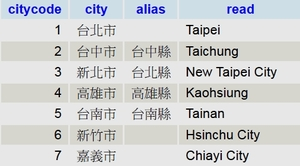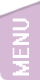[精讚] [會員登入]
3378

### [CodeIgniter 3] 資料庫的使用方法整理1/2 --Select的使用

[CodeIgniter 3] 資料庫的使用方法整理：Select的使用

#### 分享此文連結//n.sfs.tw/10173

##### 2016-11-05 20:15:01 By 張○○### 測試的資料庫結構$autoload['libraries'] = array('database'); ### 建立查詢 這是建立查詢的基本步驟$sql = "SELECT * FROM TW_city";  //這是SQL語法
$q =$this->db->query( $sql ); 這個步驟會建立mysql_query的物件，如果是Select的話再利用這個物件的方法叫回資料；如果是其他的方法如insert/update/delete則就會直接執行語法，並回傳true(達行成功)/ false(執行失敗)。 所以說，這個$q 就是建立查詢完畢後的mysql物件。

### 取得一行

1. 取得一行物件資料，這裡取得的是物件
$obj =$q->row();
印出其中一欄
print $obj->city; //台北市 若印出的物件不存在，會出現未定義物件的錯誤： print$obj->test;
出現錯誤
Notice: Undefined property: stdClass::$test 2. 取得一行陣列資料，這裡取得的是陣列$r = $q->row_array(); 印出其中一欄 print$r['city'];  //台北市
若印出的陣列索引不存在，會出現未定義索引的錯誤：
print $r['test']; 出現錯誤 Notice: Undefined index: test 3. 取得指定行--陣列用法$r = $q->row_array(3); //索引3，由0請算代表第4個 // 印出其中一欄 print$r['city'];  //高雄市

// 取得下一列
$r =$q->next_row('array');
print $r['city']; //台南市 // 取得不存在的索引$r = $q->row_array(30); //索引30，不存在，結果等於第一行：台北市$r = $q->row_array(-1); //索引-1 不存在，結果等於第一行：台北市 4. 取得指定行--物件用法$obj = $q->row(0); //索引0，由0請算代表第1個 print$obj->city;  //台北市

// 取得上一列
$obj =$q->previous_row();
print $obj->city; //台北市，到最上面，所以第0行的上一行還是第0行$obj =  $q->row(300); //索引不存在 print$obj->city;  // 台北市
$obj =$q->row(-1);  //索引不存在
print $obj->city; // 台北市 5. 如果本身就無資料$sql = "SELECT * FROM TW_city where citycode>10";  //這個SQL查詢不到任何資料
$q =$this->db->query( $sql );$obj = $q->row(); //NULL print$obj->city;  // 出現 Trying to get property of non-object 這個錯誤

$r =$q->row_array();  // NULL
if ($q->row_array() ){ ... } 5. 儘量不要指定索引位置，它會讓你搞不清楚你現在到底取第幾行，如果想指定位置，請用SQL來指定。$r = $q->row_array(); 建議$r = $q->row_array(3); 不建議指定 6. 儘量不要用這些函數，它們會讓你搞不清楚東西南北$row = $q->first_row()$row = $q->last_row()$row = $q->next_row()$row =   $q->previous_row() 7. 請在SQL上設定 limit 1，在查詢時就只取一行 ### 取得一個值 有時我們只要取得一個欄位，可使用下面這個方法取出其中的一欄$r = $q->row_array(); 印出其中一欄 print$r['city'];  //台北市

$sql ="SELECT count(city) FROM TW_city";$q = $this->db->query($sql);

$r=$q->row_array();
print array_shift($r); //就是我們要的那一欄 這樣有一個缺點，如果查詢不到任何資料，會出現 array_shift() expects parameter 1 to be array, null given 錯誤 ### 取得全部 1. 取得全部的物件寫法 foreach ($q->result() as $row) { echo$row->city;
echo $row->alias; echo$row->read;
}

2. 取得全部的陣列寫法

foreach ($q->result_array() as$r)
{
echo $r['city']; echo$r['alias'];
echo $r['read']; } ### 取得全部小結 1.$q->result() 代表著全部資料的物件；$q->result_array() 代表著全部資料的陣列； 2. 建議使用陣列寫法 ### unbuffered_row 和 row 的主要差異 前面使用的 row() ,row_array(), result(), result_array() 的方法都是先將資料庫查詢的結果放到記憶體中，然後再取出，這樣的優點是對資料庫的存取少，速度快，缺點是耗損記憶體空間。 使用 unbuffered_row 這個方法就能避免這個困難，他是循序讀取資料後，指標會指到下一筆資料，直到回傳null為止(結束)，所以對資料庫的存取就會多，但不太佔用記憶體。 所以如果大資料(例如50行以上大量數據)，請使用unbuffered_row的方法來取代 row。 ### 使用 while 在while 中只能用unbuffered_row 這個方法，因為他回傳資料後，指標會指到下一筆資料，直到回傳null為止(結束)，請勿在while中使用row()方法，會變成無窮迴圈。 1. 物件方法 while($r= $q->unbuffered_row()){ print$r->city;
}

2. 陣列方法

while($r=$q->unbuffered_row('array')){
print $r['city']; } 使用while如果資料是空值null，大括號裡的部分不會被執行 ### 使用輔助函數 1. num_rows() 取回資料庫查詢出來的行數。$q = $this->db->query($sql);
print $q->num_rows(); 2.num_fields() 取回資料庫查詢出來每行的欄數。$q = $this->db->query($sql);
print $q->num_fields(); 3. free_result() 清空查詢所有的結果，也就是這個$q 會變成空物件。原則上在php執行到最後自動會清除掉所有的變數，但是如果執行過程中要多次存取大資料，可以手動清除。

print $q->num_fields(); //4$q->free_result();
print $q->num_fields(); // 出錯 Trying to get property of non-object，因為$q已經是空物件

4.  data_seek(n)

跳過前面的 n筆資料
$q->data_seek(3); // 跳過前面的 3筆資料 while($r= $q->unbuffered_row('array')){ print$r['city'];    // 從高雄市開始印
}

如果使用2次以上，並不會影響結果

$q->data_seek(3); // 跳過前面的 3筆資料$r = $q->row_array(); print$r['city'];   //高雄市
$q->data_seek(3); // 跳過前面的 3筆資料$r = $q->row_array(); print$r['city']; //結果還是高雄市，指標還是在0的位置

5. list_fields()

傳回欄位名稱的陣列

### 整理的函數

  /****  資料庫用  ***/
// 傳回全部陣列，無資料回傳NULL
public function getAll($query){ return$query->result_array();
}
// 傳回一整欄單一陣列，無資料回傳NULL
// @param $item: 欄位名 public function getCol($query, $item=""){$all=$query->result_array();$col=array();
foreach($all as$it){
if($item &&$it[$item])$col[]=$it[$item];
if(!$item)$col[]=array_shift($it); } return$col;
}

// 傳回一個值，無資料回傳 false;
public function getOne($query){$row=$query->row_array(); if($row) return array_shift($row); return false; } //傳回一整行，無資料回傳NULL public function getRow($query){
return \$query->row_array();
}

### 參考資料

 CI3 DATABASE官網文件 http://www.codeigniter.com/userguide3/database/index.html

END

### 你可能感興趣的文章

[PHP] CodeIgniter 3+pure+smarty安裝及環境設置2/2 PHP framework CodeIgniter 3+ pure CSS +smarty Template Engine的整合#2

[CodeIgniter3] 解決無法上傳特定檔案(.sb2)的問題 上傳時出現The filetype you are attempting to upload is not allowed，要怎麼解決？

[Centos 6& 7] 安裝php-geoip php-geoip是PHP由domain、ip查詢城市國家資料非常強的函式

[PHP] IPv6檢查IP是否在某個網段內 mtachcidr6 要檢查IPv6是否在某個IPv6的網段內？

[PHP]解決ksort新增的SORT_NATURAL|SORT_FLAG_CASE方法 php>=5.4中ksort函數多了SORT_NATURAL 和 SORT_FLAG_CASE 旗標，對舊版的PHP中要怎麼辦？

>>

[無留言]

### 隨機好文